College Physics : Harmonics and Standing Waves

Example Questions

Example Question #1 : Harmonics And Standing Waves

Suppose that the fifth harmonic of a standing wave contained within a pipe closed at both ends has a wavelength of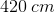. What is the length of the pipe?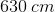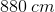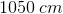Explanation:

For this question, we're told that a standing wave is contained within a pipe closed at both ends. We're also given the wavelength for the fifth harmonic, and are asked to find the length of the pipe.

The first step to solve this problem is to use the equation for a pipe closed at both ends.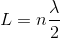Since we're told which harmonic the wave is on, as well as its wavelength, we have everything we need to solve for the length of the pipe.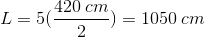Example Question #76 : College Physics

A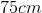string under tension is oscillated at the 5th harmonic. What is the wavelength of this oscillation?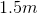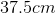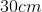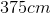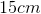Explanation:

Each harmonic has a wavelength that behaves according to the equation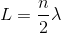, where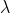is the wavelength andis the harmonic.

Since this is the 5th harmonic,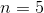and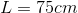. Solving for wavelength gives.

Example Question #77 : College Physics

A pipe has a length of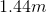. If the pipe is open at both ends, what is the frequency of the third harmonic assuming that the velocity in which sound moves through the air is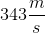.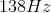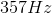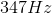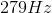Explanation:

Since the pipe is open at both ends, we can use the equation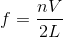where f is the frequency we are solving for,is the number of the harmonic,is the velocity at which sound moves through air, and L is the length of the pipe.

We know from the question that: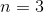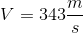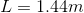When we plug in these values into the frequency equation, we getas the answer.

All College Physics Resources